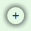# Break-Even Analysis

The Break-Even Analysis, or simply BEA, is a mathematical computation that helps a business identify the point from which it becomes profitable (break-even point). Simply put, it tells a business at what point it covered all the cost of doing business, and subsequently, starts making profits.

This break even calculator allows a business to accomplish the following:

• Determine the quantity it needs to produce or sell in order to break-even;
• Determine the selling price it needs to charge for a specific quantity you sell in order to break-even.
 Input Results Selling Price per Unit: Break-even units: 0.00 Fixed Cost: Total Revenue: \$ 0.00 Variable Cost per Unit: Total Cost: \$ 0.00Change variables Profit: \$ 0.00 Note: This calculator is a JavaScript program. You must have JavaScript enabled in order to use it.

## Definitions and terms used in the Break-Even Analysis (BEA)

• Selling Price per Unit: the price that a unit is expected to be sold for.
• Selling Units: the number of units expected to be sold (determined by a contract or market research).
• Fixed Cost (FC): the cost that remains constant within a range of production or sales, regardless of the number of units produced or sold within that range. Typical fixed costs are: rent, mortgage, equipment, salaries, insurance, fixed utilities (office utilities) etc.
• Variable Cost per Unit: the cost that vary with the production or the purchase of one unit.
• Break-even point: the point where total revenue (total sales) equal total cost.
• Total Variable Cost (VC): the cost that varies directly with the number of units produced or sold. Typical variable costs are: materials, packaging and shipping, sales commission, hourly wages, variable utilities (factory utilities) etc.

Total Variable Cost = Selling Units x Variable Cost per Unit
• Total Cost (TC): total expenses incurred in the process of producing or selling a number of units.

Total Cost (TC) = Fixed Cost (FC) + Total Variable Cost (VC)
• Total Revenue: the total sales value of the units produced or sold.

Total Revenue = Selling Units x Selling Price per Unit
• Profit: the benefits from producing or selling a number of units.

Profit = Total Revenue - Total Cost